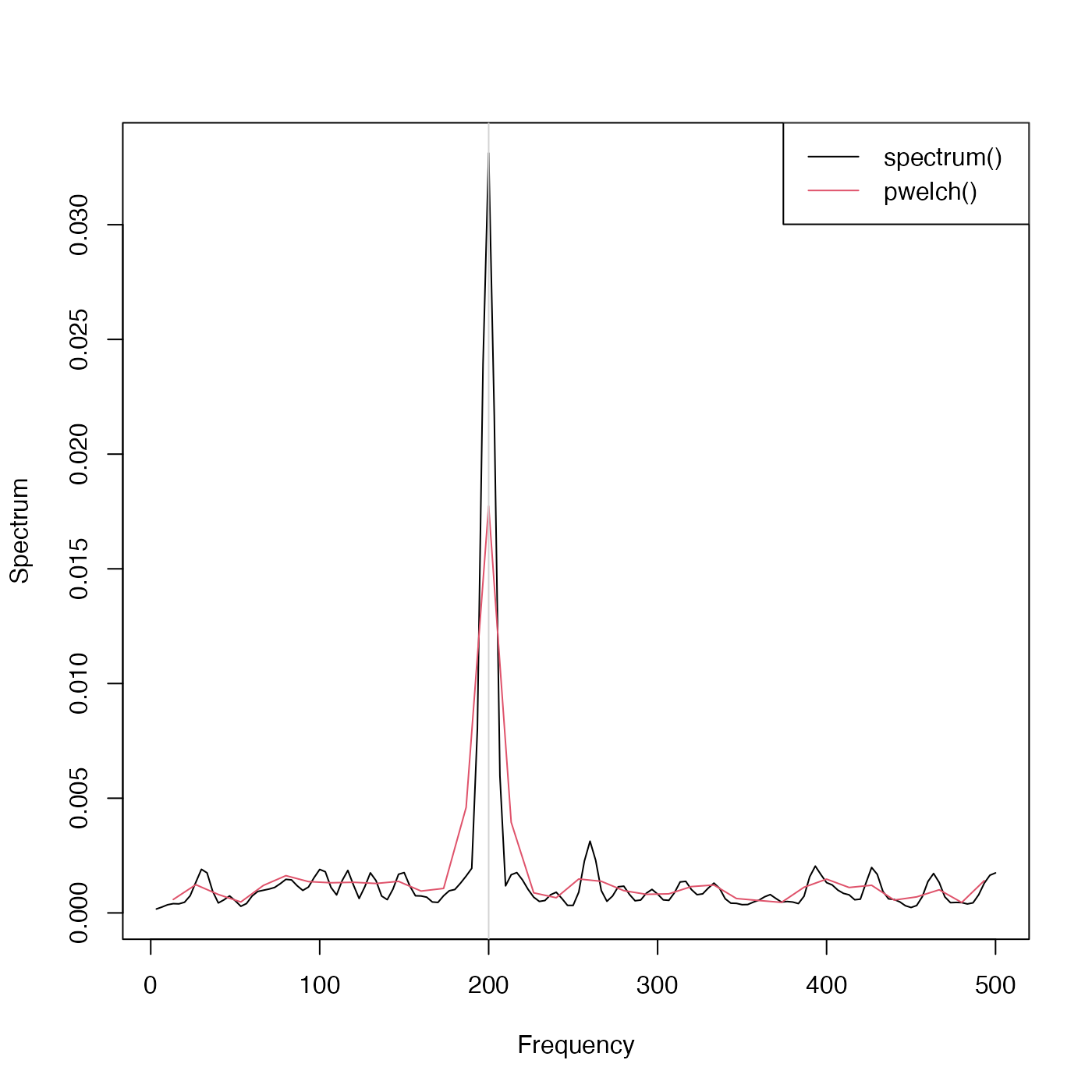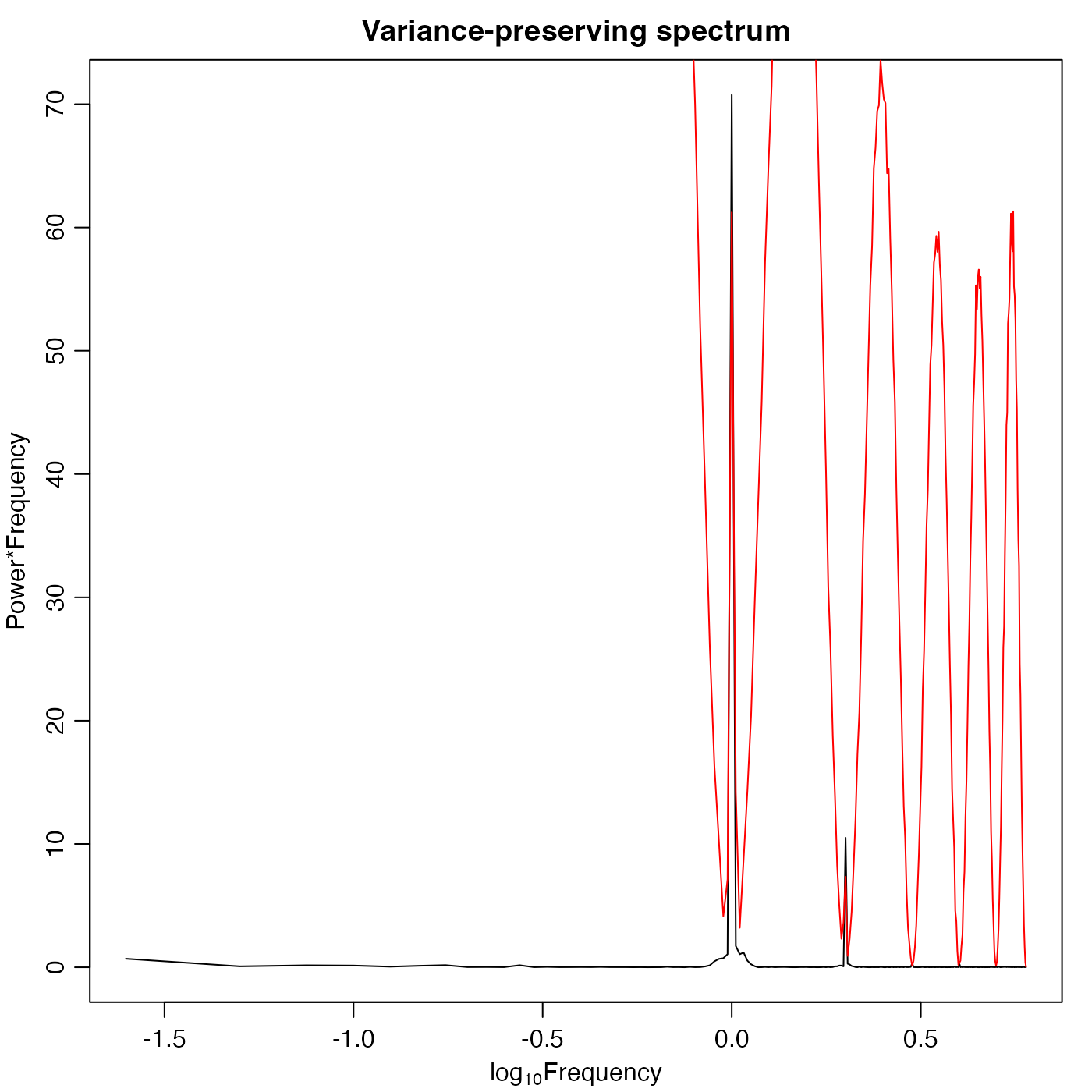Compute periodogram using the Welch (1967) method. First, x is broken up into chunks, overlapping as specified by noverlap. These chunks are then detrended with detrend(), multiplied by the window, and then passed to spectrum(). The resulting spectra are then averaged, with the results being stored in spec of the return value. Other entries of the return value mimic those returned by spectrum().

pwelch(
x,
window,
noverlap,
nfft,
fs,
spectrumtype,
esttype,
plot = TRUE,
debug = getOption("oceDebug"),
...
)

## Arguments

x a vector or timeseries to be analyzed. If a timeseries, then there is no need to specify fs. window specification, either a single value giving the number of windows to use, or a vector of window coefficients. If not specified, then 8 windows are used, each with a Hamming (raised half-cosine) window. number of points to overlap between windows. If not specified, this will be set to half the window length. length of FFT. This cannot be given if window is given, and the latter is a single integer. frequency of time-series. If x is a time-series, and if fs is supplied, then time-series is altered to have frequency fs. not used (yet) not used (yet) logical, set to TRUE to plot the spectrum. a flag that turns on debugging. Set to 1 to get a moderate amount of debugging information, or to 2 to get more. optional extra arguments to be passed to spectrum(). Unless specified in this list, spectrum() is called with plot=FALSE to prevent plotting the separate spectra, and with taper=0, which is not needed with the default Hanning window. However, the other defaults of spectrum() are used, e.g. detrend=TRUE.

## Value

List mimicking the return value from spectrum(), containing frequency freq, spectral power spec, degrees of freedom df, bandwidth bandwidth, etc.

## Bugs

Both bandwidth and degrees of freedom are just copied from the values for one of the chunk spectra, and are thus incorrect. That means the cross indicated on the graph is also incorrect.

Welch, P. D., 1967. The Use of Fast Fourier Transform for the Estimation of Power Spectra: A Method Based on Time Averaging Over Short, Modified Periodograms. IEEE Transactions on Audio Electroacoustics, AU-15, 70--73.

## Examples

library(oce)
Fs <- 1000
t <- seq(0, 0.296, 1/Fs)
x <- cos(2 * pi * t * 200) + rnorm(n=length(t))
X <- ts(x, frequency=Fs)
s <- spectrum(X, spans=c(3,2), main="random + 200 Hz", log='no')w <- pwelch(X, plot=FALSE)
lines(w$freq, w$spec, col="red")w2 <- pwelch(X, nfft=75, plot=FALSE)
lines(w2$freq, w2$spec, col='green')abline(v=200, col="blue", lty="dotted")cat("Checking spectral levels with Parseval's theorem:\n")#> Checking spectral levels with Parseval's theorem:cat("var(x)                              = ", var(x), "\n")#> var(x)                              =  1.588179 cat("2 * sum(s$spec) * diff(s$freq[1:2]) = ", 2 * sum(s$spec) * diff(s$freq[1:2]), "\n")#> 2 * sum(s$spec) * diff(s$freq[1:2]) =  1.541786 cat("sum(w$spec) * diff(s$freq[1:2])     = ", sum(w$spec) * diff(w$freq[1:2]), "\n")#> sum(w$spec) * diff(s$freq[1:2])     =  0.8662452 cat("sum(w2$spec) * diff(s$freq[1:2])    = ", sum(w2$spec) * diff(w2$freq[1:2]), "\n")#> sum(w2$spec) * diff(s$freq[1:2])    =  0.8741546 ## co2
par(mar=c(3,3,2,1), mgp=c(2,0.7,0))
s <- spectrum(co2, plot=FALSE)
plot(log10(s$freq), s$spec * s$freq, xlab=expression(log*Frequency), ylab="Power*Frequency", type='l')title("Variance-preserving spectrum")pw <- pwelch(co2, nfft=256, plot=FALSE) lines(log10(pw$freq), pw$spec * pw$freq, col='red')